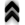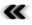### 7.3 Among Quanta

Where previous comments regarding implementations dealt with classical settings, the following will touch upon the subject of the intangible world of quantum mechanics, which was ever so brieﬂy discussed in Section 5.7. In contrast to the classical description the quantum world allows non-local coherent phenomena. The inﬂuence of these eﬀects usually diminishes, when going beyond small scales of time and space, until ﬁnally, the macroscopic world appears in its classical beauty.

#### 7.3.1 A Coherent Phase Space Illustration

The setting of quantum mechanics shall serve as an example of the application of the presented abstractions of implementation. The case of the simple inﬁnite square quantum well, illustrated in Figure 7.2, is well suited to provide an expressive example. Using Schr¨odinger’s equation (Equation 5.63) the solution to the inﬁnite square well is found to read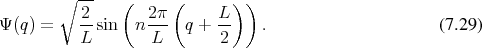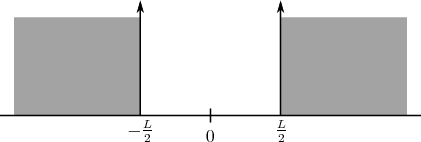Figure 7.2: Inﬁnite square quantum well.

Subjecting this analytic expression to the Wigner transformation given in Equation 5.71, again yields an analytic expression for the Wigner function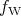deﬁned on the phase space, which takes the shape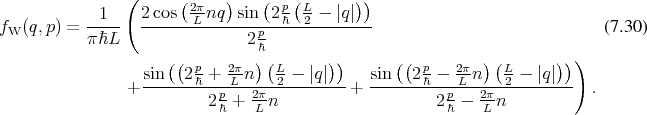As this is a phase space illustration the obtained representation now depends not only on the spatial location, but also on a momentum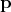as is consistent with the outlines provided in Section 5.7 and originally in Section 5.3. This expression can easily be evaluated in a point wise manner, to yield a scalar ﬁeld (Deﬁnition 61) deﬁned on the phase space. The evaluation in the discrete setting of the digital computer proceeds by iterating over all points, which represent the phase space and evaluating an expression encapsulating the prescription corresponding to Equation 7.30.

This procedure is illustrated in the following snippet of code which uses the traversal capabilities provided by the GSSE as well as functional mechanisms in the spirit of Boost Phoenix . It should be noted that the described procedure is ﬁt to be used with structured grids as well as unstructured meshes, since topology based traversal is handled by the GSSE. Furthermore, it is also important to note that the traversal of the phase space nodes is completely independent of the dimension of the phase space. The functional expression used to compute the value of the scalar ﬁeld needs to be able to deal with the dimensionality it is provided with. In this particular case the analytical prescription to obtain the phase space description corresponding to a particle in the inﬁnite square quantum well can be extended to, in theory, arbitrary dimensions, albeit visualization of the resulting phase spaces is no longer easily possible.

auto const cwf = calc_wigner_function<numeric_t>(L, order);

gsse::traverse()

std::cout << cwf( gsse::acc0 , gsse::acc1 ) << std::endl
]( phase_space );

The results obtained in this fashion are illustrated in Figure 7.3 and Figure 7.4 for diﬀerent values of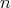, corresponding to diﬀerent Eigenstates.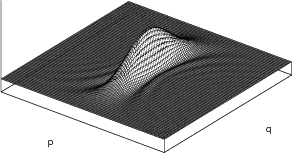Figure 7.3: Wigner function in the phase space corresponding to the ﬁrst level (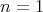) in an inﬁnite square quantum well.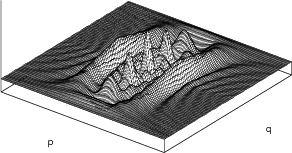Figure 7.4: Wigner function in the phase space corresponding to the seventh level (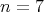) in an inﬁnite square quantum well.

#### 7.3.2 Phase Space Capturing Quantum Eﬀects

The bound states of a contained particle already are profound expression of quantum behaviour. Quantum mechanics using wave functions, however, allows for additional eﬀects. The following example shall illustrate that such eﬀects also ﬁnd their expression, when using the phase space description. To this end a particle which is represented by a wave packet of the following form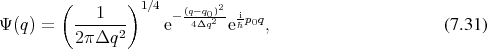where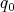and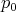are the central position and the mean momentum respectively and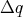represents the width of the wave packet, shall be considered. Subjecting Equation 7.31 to the Wigner transform (Equation 5.71) readily yields the phase space representation which can be given analytically asAgain using the traversal capabilities of the GSSE, the evaluation of this expression on the phase space is identical to the previous case with the exception of supplying an appropriate functor in accordance with Equation 7.32. Figure 7.5 provides an illustration of this wave packet in phase space, thus depending on both location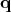and momentum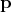, centred at the origin.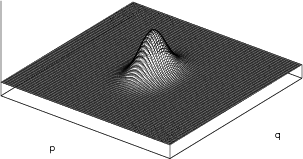Figure 7.5: Phase space representation of a wave packet.

Moving beyond a single particle and thus a single wave packet, two particles in proximity to one another are considered. Proceeding by simply adding the two pseudo density functions resulting from the wave packets, a result as in Figure 7.6 is obtained, which corresponds to classical expectations regarding the interactions of particles.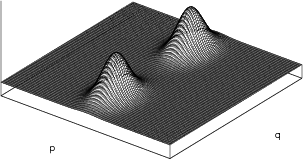Figure 7.6: Two distinct wave packets placed symmetrically around the origin.

The quantum mechanical setting, however, also allows for a diﬀerent case, which can be explored by adding the wave functions comprising the two individual wave packets before applying the transformation to phase space. Proceeding in this manner yields the expression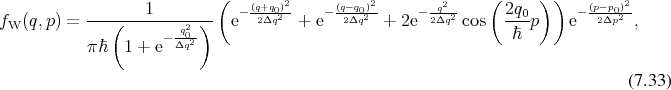which, encompasses additional features, as can be seen in Figure 7.7 which has again been obtained using the traversal scheme. Markedly diﬀerent characteristics when compared to Figure 7.6 are clearly observable in between the two wave packets, which represent a feature of quantum mechanics.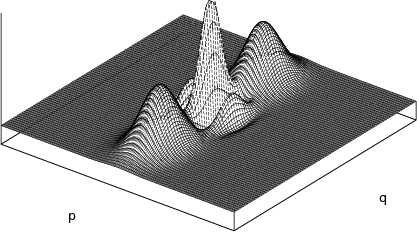Figure 7.7: Two wave packets interacting.